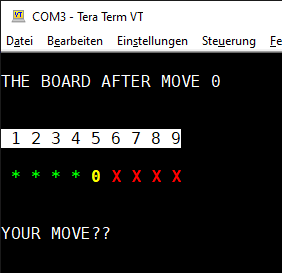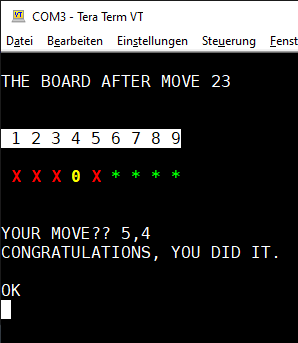# a BASIC Quicky

Merry Christmas Folks,
🎄🎅🍷🍷🍷

And with these 4 (!) days off, I really recovered some of my health problems. The flu I cought was the toughest thing I had in 20 years.
But now I'm back on track and ready to spend my time on important things, not.

Here is a short BASIC Puzzle I've found in Kilobaud 11-78: Lucas' Puzzle by William L. ColsherRemarks: I picked Dave Hasslers ideas and used ANSI sequences to bring in some color. Also there was a non working round counter I fixed.

``````5 REM Using ANSI ESC sequences for some color...
6 E\$=CHR\$(27)+CHR\$(91):CL\$=E\$+"2J":HM\$=E\$+"H"
7 GRN\$=E\$+"1;32m":YLW\$=E\$+"1;33m"
8 RV\$=E\$+"7m":N\$=E\$+"m":RED\$=E\$+"1;31m"
9 POKE 10920,169 :REM CXL <RETURN> BREAK ON INPUT
10 INPUT "INSTRUCTIONS?";I\$
20 IF I\$ = "Y"THEN GOSUB 500
30 DIM B(9)
40 FOR I = 1 TO 4
50 LET B(I)=1
60 NEXT I
70 LET B(5)=0
80 FOR I=6 TO 9
90 LET B(I)=10
100 NEXT I
109 LET M=0
110 REM TEST
120 PRINT CL\$ : PRINT HM\$
121 PRINT "THE BOARD AFTER MOVE";M
130 PRINT ""
140 PRINT ""
150 PRINT RV\$+" 1 2 3 4 5 6 7 8 9"+N\$
160 PRINT ""
170 FOR I = 1 TO 9
180 IF B(I)=0 THEN PRINT YLW\$" 0";
190 IF B(I)=1 THEN PRINT GRN\$+" *";
200 IF B(I)=10 THEN PRINT RED\$+" X";
210 NEXT I
220 PRINT N\$+""
230 PRINT ""
250 IF X=99 THEN GOTO 760
260 GOSUB 350
270 IF F=1 THEN GOTO 240
280 LET B(Y)=B(X)
290 LET B(X)=0
295 M=M+1
300 FOR I=1 TO 4
310 IF B(I)<>10 THEN 340
320 NEXT I
330 IF B(5)=0 THEN GOTO 770
340 GOTO 110
350 LET F=0
360 IF B(Y) <> 0 THEN GOTO 470
370 IF B(X) = 10THEN GOTO 440
380 IF B(X) = 1 THEN GOTO 410
390 IF B(X) = 0 THEN GOTO 470
400 GOTO 470
410 IF X >=Y THEN GOTO 470
420 IF Y-X > 2 THEN GOTO 470
430 RETURN
440 IF X <=Y THEN GOTO 470
450 IF X-Y > 2 THEN GOTO 470
460 RETURN
470 PRINT "ILLEGAL MOVE. REENTER."
480 LET F=1
490 RETURN
500 PRINT "" : PRINT ""
510 PRINT "LUCAS' PROBLEM"
520 PRINT "" : PRINT ""
530 PRINT "THE OBJECT OF THIS GAME IS TO"
540 PRINT "EXCHANGE THE POSITIONS OF THE "
550 PRINT "TWO SETS OF MARKERS; * AND X"
560 PRINT "TO DO THIS YOU MUST MOVE THEM"
570 PRINT "INTO THE SPACE MARKED WITH AN"
580 PRINT " '0'. THE MARKERS MAY BE MOVED"
590 PRINT "EITHER INTO THE '0' SPACE"
600 PRINT "IF IT IS ADJACENT OR A SINGLE"
610 PRINT "MAKER MAY BE JUMPED."
620 PRINT "THE '*' MARKERS MOVE ONLY"
630 PRINT "TO THE RIGHT AND THE 'X' ONES"
635 PRINT "ONLY TO THE LEFT."
640 PRINT ""
670 PRINT "" : PRINT"A MOVE IS MADE BY ENTERING THE"
680 PRINT "NUMBER ABOVE THE MARKER TO BE MOVED"
690 PRINT "AND THE NUMBER OF THE SPACE"
700 PRINT "TO WHICH IT IS TO BE"
710 PRINT "MOVED. THE PUZZLE CAN BE SOLVED"
720 PRINT "IN 24 MOVES."
730 PRINT ""